Order Form Medical / Limited
Group Orders Allowed.
Each patient must submit certification.

1 pound maximum per order.
•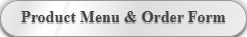• Submit medical cannabis certifications here.
• - -
•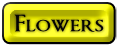•12.0% to 14.9% THC
• \$0.38 to \$0.44 cents per gram
• \$.93 cents to \$2.85 per gram
• \$.93 cents to \$2.85 per gram
• \$.93 cents to \$2.85 per gram
• \$.93 cents to \$2.85 per gram
•Producers Special
\$195.00 a pound
• \$.43 cents to \$.55 cents per gram
•15.0% to 17.9% THC
• \$1.64 to \$4.28 per gram
• \$1.64 to \$4.28 per gram
• \$1.64 to \$4.28 per gram
• \$1.64 to \$4.28 per gram
• \$1.64 to \$4.28 per gram
• \$1.64 to \$4.28 per gram
•Producers Special
\$530.00 a pound
• \$1.16 to \$1.43 per gram
• \$1.16 to \$1.43 per gram
•18.0% to 20.9% THC
• \$2.10 to \$4.64 per gram
• \$2.10 to \$4.64 per gram
• \$2.10 to \$4.64 per gram
• \$2.10 to \$4.64 per gram
• \$2.10 to \$4.64 per gram
• \$2.10 to \$4.64 per gram
• \$2.10 to \$4.64 per gram
• \$2.10 to \$4.64 per gram
• \$2.10 to \$4.64 per gram
• \$2.10 to \$4.64 per gram
• \$2.10 to \$4.64 per gram
• \$2.10 to \$4.64 per gram
• \$2.10 to \$4.64 per gram
• \$2.10 to \$4.64 per gram
• \$2.10 to \$4.64 per gram
•Producers Special
\$980.00 a pound
• \$2.15 to \$2.42 per gram
•Producers Special
\$950.00 a pound
• \$2.09 to \$2.42 per gram per gram
•21%+ THC
• \$2.56 tp \$8.57 per gram
• \$2.56 to \$8.57 per gram
• \$2.56 to \$8.57 per gram
• \$2.56 to \$8.57 per gram
• \$2.56 to \$8.57 per gram
• \$2.56 to \$8.57 per gram
• \$2.56 to \$8.57 per gram
• \$2.56 to \$8.57 per gram
• \$2.56 to \$8.57 per gram
•Producers Special
\$1,060.00 a pound
• \$2.33 to \$\$2.62 per gram
•Producers Special
\$1,050.00 a pound
• \$2.31 to \$2.62 per gram
•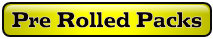• \$6.18 to \$6.33 per gram
• \$6.18 to \$6.33 per gram
• \$6.18 to \$6.33 per gram
• \$6.18 to \$6.33 per gram
• \$7.96 to \$16.25 per gram
• \$19.00 to \$35.00 each
• \$19.00 to \$35.00 each
• \$6.18 to \$6.33 per gram
• \$20.75 to \$35.00 per gram
• \$20.75 to \$35.00 per gram
• \$20.75 to \$35.00 per gram
•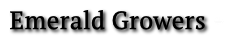• \$5.95 to \$8.21 each
• \$4.35 to \$6.78 each
• \$7.16 to \$9.28 each
• \$5.95 to \$8.21 each
•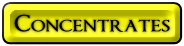• \$3.98 to \$10.71 per gram
• \$7.15 to \$15.00 per gram
• \$4.64 to \$10.00 per gram
• \$7.93 to \$15.00 per gram
• \$8.26 to \$15.71 per gram
• \$1.88 to \$5.42 per gram
• \$5.72 to \$15.00 per gram
• \$5.16 to \$16.42 per gram
• \$7.14 to \$15.71 per gram
• \$3.30 to \$15.00 per gram
•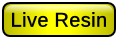One Ounce Jars
•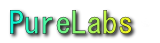\$24.80 to \$36.66 per gram
•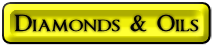•• \$40.00 to \$55.00 per gram
•• \$45.35 to \$61.66 per gram
• \$16.25 to \$23.33 per gram
•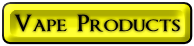C Cell
•• One gram oil each
• One gram oil each
• One gram oil each
• One gram oil each
• One gram oil each
• One gram oil each
• One gram oil each
• One gram oil each
• One gram oil each
• One gram oil each
• One gram oil each
• One gram oil each
• One gram oil each
•C Cell
• One gram oil each
• One gram oil each
• One gram oil each
• One gram oil each
• One gram oil each
• One gram oil each
• Vape Pen with charger
•Cartridges
•C Cell
•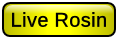•C Cell
• ### Cosmos Brand

Mushroom
100% organic natural flavors
•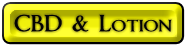•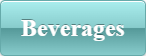Nine (9) bottles maximum per order
without Membership
• ### Fun Drinks

\$15.00 per bottle
• ### Rum

\$30.00 per bottle
• 5ML Nano 21% HHC 19% D8
• ### Red Wine

\$45.00 per bottle
• 5ML Nano 21% HHC 19% D8
•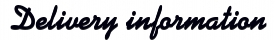• ### All orders must be paid in full in advance.

Depending upon your location, delivery time varies from 2, up to 5 days, in extreme cases, or delays from extreme weather conditions.
• If you have one.
• Total weight
• *USPS money order may delay delivery up to 2 days.
They can be difficult to cash.